# Carbon dioxide (CO2) lewis dot structure, molecular geometry, bond angle, hybridization, electron geometry

Home  > Chemistry Article > CO2 lewis structure and its molecular geometry/shape

###Carbon dioxide is made up of one hydrogen and two oxygen connected with a double bond with the chemical formula CO2. It is a combustible gas resulting from the oxidation of carbon.

In this article, we will discuss Carbon dioxide (CO2) lewis structure, molecular or electron geometry, hybridization, bond angle, polar or nonpolar, etc.

At room temperature, carbon dioxide normally acts as gas but at high pressure, it turned into liquid. CO2 can be an environmental disaster due to its heat-absorbing properties.

CO2 is normally present in our blood at a normal range but a large dose of it is very toxic to the human body or other living organisms.

Useful properties of Carbon dioxide

• It is heavier than air.
• It is soluble in water.
• In high concentrations, its odor becomes acidic.
• It has a molar mass of 44.009 g·mol−1.
• The melting point of CO2 is −56.6 °C.
• Relatively it is nontoxic and noncombustible.
• CO2 can make bubbles in soft drinks.
• Carbon dioxide turns lime water into milky.
 Name of Molecule Carbon dioxide Chemical formula CO2 Molecular geometry of CO2 Linear Electron geometry of CO2 Linear Hybridization Sp Bond angle 180º Total Valence electron for CO2 lewis structure 16 The total formal charge of CO2 0
Page Contents

## How to draw Carbon dioxide (CO2) lewis structure?

CO2 lewis structure contains two oxygen atoms and one carbon atom, connected with the double bond whereas carbon is the central atom, and no lone pair is present on it.

But each oxygen in the CO2 lewis dot structure has two lone pairs. A lewis diagram helps us to know how electrons are arranged around individual atoms in a molecule.

Let’s see how to draw a CO2 lewis structure with simple steps.

## Follow some steps for drawing the CO2 Lewis dot structure

### 1. Count total valence electron in CO2

In the first step, we need to calculate how many valence electrons are present in it. Valence electrons of CO2 show the outer shell electrons present around carbon and oxygen that can participate in the formation of the chemical bonds.

Valence electrons of Carbon and Oxygen are represented as dots in the lewis diagram. To find the valence electron of carbon and oxygen we need the help of a periodic table.

By looking at the periodic table, we come to know carbon belongs to 14 groups and oxygen belongs to the 16th group in the periodic table. Hence, carbon has 4 valence electrons and oxygen has 6 valence electrons.

Valence electron of Oxygen = 6          [∴Periodic group of oxygen = 16]

Valence electron of Carbon = 4          [∴Periodic group of carbon = 14]

Total valence electron available for drawing the CO2 lewis structure = 4 + 2*6 = 16 valence electrons### 2. Find the least electronegative atom and placed it at center

Now we need to find which atom(Carbon or oxygen) has the least electronegativity then placing that atom to the center in lewis’s diagram. Electronegativity increase from left to right in the periodic table.

As carbon belongs to the 14th group which is at the left and oxygen belongs to the 16th group which is at the right in the periodic table.

Clearly, carbon is less electronegative than oxygen, therefore, place it at the center in the lewis diagram and oxygen spaced evenly around it.### 3. Connect carbon and oxygen with a single bond

In the third step, we will start to draw the lewis structure of CO2 by connecting the outer atom(Oxygen) to the central atom(Carbon) with the help of a single bond.By looking at the above diagram, we come to know two single bonds are used that contain 4 electrons. So, we used 4 electrons from a total of 16 valence electrons that are available for drawing the Lewis structure of CO2.

∴ (16 – 4) = 12 valence electrons

Now we are left with 12 valence electrons.

### 4. Placed remaining valence electrons around the outer atom

As we are left with 12 valence electrons and we have to place these electrons around the outer atom(Oxygen) first to complete its octet rule. “Octet rule show that atom is most stable when eight electrons present in its valence shell.”

So, oxygen needs 8 electrons around it for coming into the stable zone. Therefore, place the remaining valence electron around oxygen first for completing its octet shell.So, look at the above diagram and see how many valence electrons we used till now and how many are left. Each oxygen has 8 electrons(6 dot electrons + 2 electrons in a single bond) therefore oxygen completes its octets comfortably.

In the above diagram, 16 valence electrons are used(6 on each oxygen atom + 4 electrons in form of two single bonds)

### 5. Complete central atom octet and make covalent bond if necessary

Now, this is the final step for completing the CO2 lewis dot structure. In this step, we have to complete the central atom(Carbon) octet for its stability.

As carbon needs 8 electrons to complete its octet shell but carbon has only 4 electrons(two single bonds) around it and it needs 4 more to complete its octet.

Also, we have no extra valence electrons left for completing the octet of carbon. So, to overcome this problem, we will take the help of oxygen lone pair electrons.

Take two lone pair electrons from each oxygen and convert them into a covalent bond.### Carbon dioxide (CO2) lewis structure

Now look at the above CO2 lewis dot structure and see if the atoms of CO2 completed their octet or not. Yes, both atoms(Carbon and oxygen) have completed their octet rule comfortably as each of them has 8 electrons in the outermost shell.

### 6. Check the stability with the help of a formal charge concept

Now just check the stability of the CO2 lewis structure with the help of the formal charge concept.

“The lesser the formal charge on atoms, the better is the stability of the lewis diagram.”

To calculate the formal charge on an atom. Use the formula given below-For carbon atom:

⇒ Valence electrons of carbon = 4

⇒ Lone pair electrons on carbon = 0

⇒ Shared pair electrons around carbon(two double bonds) = 8

∴ (4 – 0 – 8/2) = 0 formal charge on the central carbon atom.

For oxygen atom

⇒ Valence electrons of oxygen = 6

⇒ Lone pair electrons on oxygen = 4

⇒ Shared pair electrons around oxygen (one double bond) = 4

∴ (6 – 4 – 4/2) = o formal charge on the oxygen atom.

So, both atoms(carbon and oxygen) get the formal charge zero.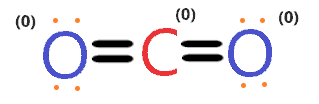Therefore, the above lewis structure of CO2 is better and most stable as the overall formal charge is zero.

## What is the electron and molecular geometry of CO2 (Carbon dioxide)?

Molecular geometry is an arrangement of atoms in a molecule. CO2 lewis structure can give us an approximate measure of its molecular shape but to determine the precise molecular geometry of CO2, we need to look at the VSEPR theory.

VSEPR theory predicts the shape of the molecule by taking the measure of repulsion between electron pairs in the valence shell. Two types of geometry can be predicted with the help of this theory- (a). Electron geometry (b). Molecular geometry

Electron geometry considers all electrons(Bonding and Antibonding electrons) whereas molecular geometry considers only Bonding electrons to determine the shape of any molecule.

Now the question arises what is the molecular geometry or shape of CO2? The molecular geometry of CO2 is linear because the valence shell electron pairs present around the carbon central atom and lone pairs on both sides oxygen atoms will repel each other, as a result, repulsion on the left and the right sides is the same as carbon central atom is doubly bonded with each oxygen and no lone pair present on the central atom.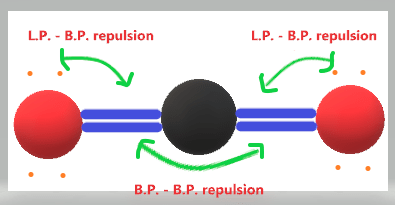As you see in the above figure, lone pairs on the outer atoms repelling the adjacent bonded pairs, and bonded pairs electrons repelling the other side of bonded pair electrons, all these repulsions have the same effect on both sides because the central atom has zero lone pair, so, it stretches the structure in a straight line.

For keeping the repulsive force least generated by the bonding pair of electrons and lone pair on the outer atoms, CO2 acquires the linear molecular shape.

The electron geometry of CO2 is also linear because there is no lone pair present on the central atom that can cause disorientation in the molecule.

Now you know the molecular and electron geometry of CO2 but how to find it theoretically?

## Follow three steps to find CO2 molecular shape and its electron geometry

### 1. Find the Number of lone pairs present on the central atom of the CO2 Lewis structure

As we know carbon is the central atom in the CO2 lewis structure and it has no lone pair of electrons but each oxygen has two lone pairs of electrons.

Or you can determine the lone pair with the help of the formula given below-

L.P = (V.E. – N.E.)/2

where L.P. = Lone pair on the central atom

⇒ V.E. = valence electron of that central atom

⇒ N.E. = Number of electrons taken part in bonding with the central atom

So, the central atom is carbon, and the number of valence electrons of carbon is 4 with four bonding electrons attached to it.

Put all these values in the given formula-

∴ (4 – 4)/2

= o is the lone pair present on the central atom of the CO2 lewis dot structure.

### 2. Find hybridization number of CO2

Hybridization number is very helpful to determine the molecular geometry or shape of CO2. According to the VSEPR theory, a Steric number is used to determine the hybridization of an atom.

When the Steric number is equal to 2 then the hybridization of that atom is sp and if it is equal to 3 then Sp²…..so on.

Note: Steric number = Hybridization number

The formula for finding the steric number of atoms.

S.N. = N.A. + L.P.

where S.N. = Steric number of atom

⇒ N.A. = Number of atoms attached to the central atom

⇒ L.P. = lone pairs on that central atom

According to CO2 lewis’s structure, Carbon is the central atom that has zero lone pair on it and two-atom(Oxygen) attached to it.

Therefore, S.N. = 2 + 0

∴ S.N. = 2

So, two steric number means carbon has Sp hybridization.

You can also find the steric number of the Oxygen atom. As each oxygen in the electron dot structure of CO2 has two lone pairs on it and is attached to 1 Carbon atom.

S.N. of Oxygen = 2 + 1

= 3 is the steric number of oxygen that means each oxygen has Sp² hybridization.But to determine the molecular geometry of CO2 we can use the hybridization of the central atom(Carbon) only.

### 3. Use VSEPR theory or AXN method to determine CO2 molecular shape/geometry

Now we have two methods to determine the molecular or electron geometry of CO2. (a). VSEPR theory (b). AXN method

According to the VSEPR theory, if any molecule contains zero lone pair and Sp hybridization then the molecular geometry of that molecule will be linear and electron geometry also linear.

So, the molecular shape of the CO2 is linear as it has o lone pair and Sp hybridization.

### CO2 molecular geometry or shape

Now the second way to determine the molecular geometry of CO2 is the AXN method.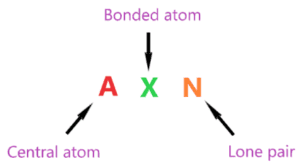According to this method-

• A represents the central atom.
• X represents the bonded pairs of electrons to the central atom.
• N represents the lone pairs of electrons on the central atom

So, Carbon is the central atom that has two bonded atoms and 0 lone pairs on it.

Hence formula of CO2 becomes AX2.

According to the VSEPR chart, if any molecule has the AX2 formula then the molecule geometry of that molecule is linear and electron geometry is also linear.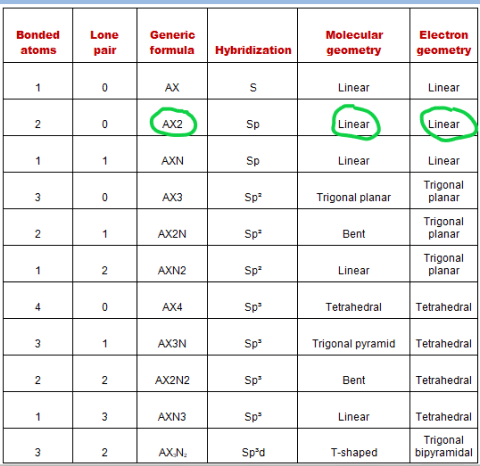The bond angle of CO2 is 180º as expected because the carbon atom attains Sp hybridized and no lone pair present on it. Therefore, no disorientation occurs around the central atom which makes it’s linear in shape that has a bond angle of 180º.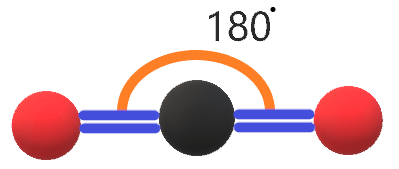## Carbon dioxide polarity: Is CO2 polar or nonpolar?

Well, we know the polar molecule has some dipole moment because of unequal distribution of charges whereas the non-polar molecule has an equal distribution of charges that cause zero dipole moment because they cancel out each other due to the symmetrical shape of the molecule.

Is Carbon dioxide (CO2) polar or non-polar? CO2 is a non-polar molecule because it contains two bonds(C-O) that are arranged symmetrically. Due to this, dipole moment generated on both sides along C-O cancels out each other making it’s a non-polar molecule.

Let’s understand whether carbon dioxide is polar or non-polar in detail.

## Three factors that indicate the polarity of CO2

### 1. Electronegativity:

It directly influences the polarity nature of any atom or molecule. Because the polarity is directly proportional to the difference of electronegativity created by atoms or molecules. Electronegativity means the tendency of an atom to attracting electrons towards itself.

If the electronegativity difference between the atoms is high then the polarity will also be higher. Now, look at the electronegativity of carbon and oxygen.

As carbon electronegativity is around 2.6 and for oxygen, it is around 3.45. Therefore oxygen has a higher tendency to attract an electron to itself than carbon.

Also, the electronegativity difference between carbon and oxygen is more than 0.5, and according to the Pauling scale if the electronegativity difference between atoms is higher than 0.5 then the bond between that atoms behaves as polar.

But it doesn’t necessary if some bond is polar then the whole molecule can also be polar. In the case of CO2, the C-O bond is polar in nature due to the difference in electronegativity between them. But the whole molecule of CO2 is non-polar in nature.

### 2. Dipole moment

Dipole moment ensures the strength of polarity between carbon and oxygen atom. As greater the dipole moment of the molecule, the more is the polar nature of that molecule. The electronegativity difference between the atoms(Carbon and oxygen) induced positive and negative charges.

As oxygen is more electronegative than carbon hence some negative charge is induced around oxygen and a partial positive charge is induced around carbon. So, these charges create two dipole moments around C–O bond. Let’s see the figure given below.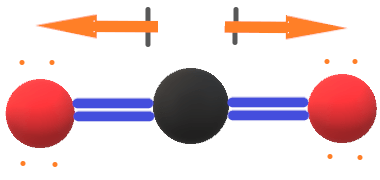As dipole moment induced in the direction from carbon to oxygen. Because oxygen is more electronegative than carbon so it attracts electrons towards itself. But dipole moment generated in between the atoms can easily be canceled out each other because the lewis structure of CO2 has linear molecular geometry that does not contain any lone pair which prevent the repulsion between bond pair of electrons.

In mathematical terms, we can express dipole moment as D = Q×R

Dipole moment formula = charge on the atoms * the distance between them.

### 3. Geometrical or molecular shape:

The geometrical structure of any molecule has great influences on the polarity nature. As the CO2 molecular geometry is linear that has two polar covalent bonds (C-O) but they are in the opposite direction. Also, a central atom of CO2 doesn’t contain any lone pair which makes its molecule shape highly symmetric.

So, the C-O bond is highly symmetric and opposite in direction which can easily be canceled out each other causing this molecule to become non-polar in nature.

## Uses of Carbon dioxide.

1. It is used as a fire extinguisher gas.
2. It is used as an inert gas in welding.
3. It can be used to freeze the food and to control the chemical reaction.
4. It is used for various purposes like as a solvent, an anesthetic, an antagonist, etc.
5. It is used as dry ice to cool things.
6. It is used to increase the speed of hardening the concrete.
7. It is used to make fizzy drinks.
8. CO2 is used for chemical production, petroleum production, and agriculture practices, etc.
9. Carbon dioxide is used to carbonate sodas.
10. It is used as a feedstock for the synthesis of chemicals and fuels.

## How Carbon dioxide is produced?

• It is produced by burning fossil fuels.
• Except for pure hydrogen, burning anything which has carbon in it like wood, plastic, gasoline, coal, etc. emits Co2.
• It can be produced by the respiration of plants and animals.
• The volcanic eruption produced Carbon dioxide.
• The decomposition of organic matter produced CO2 and various other gases like methane.
• CO2 is exhaled from our body because it is the waste product that is absorbed in our blood.

## Preparation of Carbon dioxide

(a). In laboratory preparation, CO2 is produced by reacting dilute acid with metallic carbonate.

⇒ CaCO3+2HCl  CaCl2+CO2+H2O

(b). By thermal decomposition of limestone at a temperature around 850ºC.

CaCO3 → CaO + CO2

(c). Combustion of carbon-based fuel. Example( methane reacting with oxygen).

⇒ CH4 + 2O2 → CO2 + 2H2O

(d). It is produced with the help of the fermentation of sugar.

⇒ C6H12O6 + 6O2 → 6CO2 + 6H2[Source]

## FAQ

### How many lone pair and bond pair electrons do Carbon and Oxygen have in the CO2 lewis structure?

Each oxygen in the CO2 lewis dot structure contains four lone pairs of electrons and is attached with 4 bonding pairs of electrons(one double bond contains 4 electrons) whereas the carbon atom has zero lone pairs and 8 bonding pairs of electrons.

### How many valence electrons are available for drawing the CO2 lewis dot structure?

⇒ Valence electron available for Carbon = 4

⇒ Valence electron available for Oxygen = 6

∴ Total Valence electron available for CO2 lewis dot structure = 4 + 2×6 = 16 electrons

### Why CO2 is non-polar?

CO2 is non-polar because of its symmetrical geometry and the dipole moment generated along with the C-O bond also canceled out each other as the molecular shape of CO2 is linear and it has Sp hybridization with a bond angle of 180º. Also, no lone pair is present on the central atom in the CO2 lewis structure that helps to avoid distortions in the molecule.

### Why electron and molecular geometry of CO2 are same?

As we know the molecular geometry of CO2 is linear and electron geometry is also linear because electron geometry predicts the shape of a molecule with the help of lone pair as well bond pair but molecular geometry has only taken bond pair to predict the shape of the molecule.

As there is no lone pair present on the central atom in the CO2 lewis dot structure. Therefore, both molecular and electrons geometry predict the shape of CO2 with the help of bonded pair of electrons.

Hence, the molecular geometry and electron geometry of CO2 is the same.

## Summary

Finally after a long discussion about the CO2 lewis structure or electron dot structure, molecular geometry, polarity, bond angle, etc. We are going to take the last overview of this article with the help of some bullet points.

• The net dipole moment of CO2 is zero.
• The electron and molecular geometry of CO2 are linear.
• The bond angle of CO2 is 180º and the hybridization of it is Sp.
• The total valence electron available for the CO2 lewis structure is 16.
• The overall formal charge in the CO2 lewis dot structure is zero.
• Carbon dioxide is a non-polar molecule.
##### Subscribe to Blog via Email

Join 2 other subscribers

Share it...